A non-abeliann nonlinear Schr Ìodinger equation and countable superposition of solitons 1 | OMICS International
Journal of Generalized Lie Theory and Applications

# A non-abeliann nonlinear Schr ̈odinger equation and countable superposition of solitons 1

Cornelia SCHIEBOLD*

Department of Mathematics, Mid Sweden University, S-851 70 Sundsvall, Sweden

*Corresponding Author:
C. Schiebold
Department of Mathematics Mid Sweden
University, S-851 70 Sundsvall, Sweden
E-mail: [email protected]

Received date: December 17, 2007; Revised date: March 27, 2008

Visit for more related articles at Journal of Generalized Lie Theory and Applications

#### Abstract

We study solutions of an operator-valued NLS and apply our results to construct countable superpositions of solitons for the scalar NLS.

#### Introduction and main results

In the present note we explain how to construct solutions of the NLS via the study of a corresponding non-abelian system. Non-abelian integrable systems are a very active field of recent research (cf [5, 8,10,11] to mention only a few closely related to our work). From our point of view, they provide the appropriate framework to apply functional analytic techniques to the original scalar equations. The operator theoretic part of this note is based on , where the whole AKNS is treated uniformly. We point out several sharpenings which become possible for the individual equation at hand.

Concerning applications, we have to restrict to a concise discussion of the countable superpositions of solitons. This topic was initiated by Gesztesy and collaborators with results for several other equations [6,7]. Their approach requires involved computations along the lines of ISM. Our method leads to much shorter arguments as hard analysis is replaced by advanced functional analysis. For more applications, like the asymptotic description of multipole solutions, the reader is referred to .

Replacing u by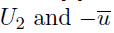by U1 in the scalar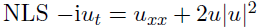yields a non-abelian NLS system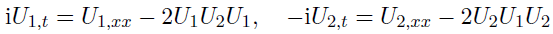(1.1)

We interpret U1, U2 as functions depending on the real variables x, t with values in the spaces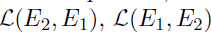of bounded linear operators mapping between Banach spaces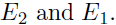In Section 2, we find soliton-like solutions of (1.1).

Theorem 1. Let E1, E2 be Banach spaces and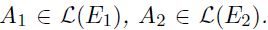Assume that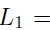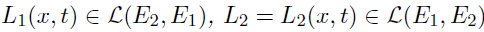are differentiable operator-functions solving the base equations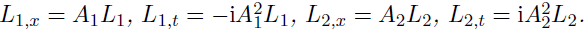Then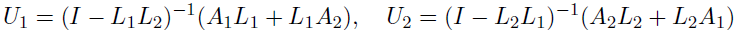(1.2)

solve the non-abelian NLS system (1.1) wherever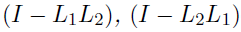are both invertible.

Since the proof of this result is mainly algebraic and does not use specific properties of bounded operators, more general formulations for functions with values in appropriate algebras are possible. We stated the above version for sake of concreteness. The special form of the solution (1.2) was obtained as a consequence of more general considerations about the noncommutative AKNS system in . A reformulation closer to the familiar scalar solution is given in Theorem 3.

In Section 3 we derive solution formulas for the NLS which still depend on arbitrary operator parameters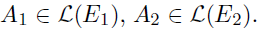Such solution formulas are actually very general (see [2,4] for the KdV case). As a concrete application, we construct countable superpositions of solitons in Sec. 4.

Theorem 2. Let (kj)j be a bounded sequence with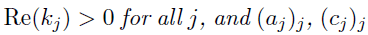sequences satisfying the growth condition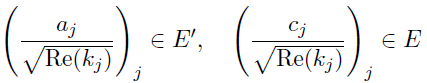(1.3)

where E is one of the classical Banach spaces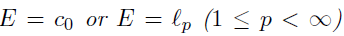and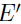is its topological dual. Then the following spectral determinants of the infinite matrices are well-defined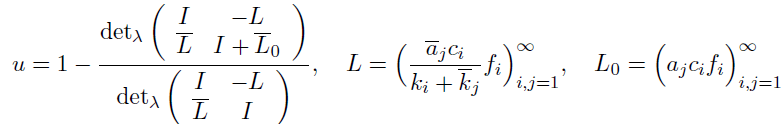where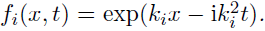Moreover, u is a solution of the NLS equation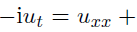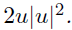If we truncate sequences by requiring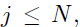the formula of Theorem 2 describes an Nsoliton uN. Then u is their limit for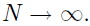Notice that neither the existence nor the solution property of u are clear a priori.

#### An operator equation governing the NLS

Let E be a Banach space and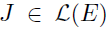with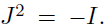For an operator-valued function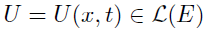we consider the non-commutative partial differential equation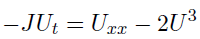(2.1)

and show that it has a traveling wave solution.

Theorem 3. Let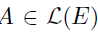with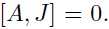Assume that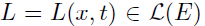is an operatorvalued function anti-commuting with J and solving the base equations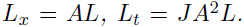Then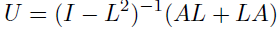(2.2)

solves the operator equation (2.1) wherever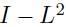is invertible.

For the proof we introduce in addition the operator-valued function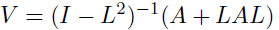(2.3)

Lemma 1. The derivative of the operator-valued functions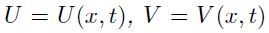given in (2.2), (2.3) with respect to x is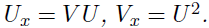Proof. First we recall the non-abelian differentiation rule for inverse operators. If T = T(x) is differentiable with respect to x and invertible for all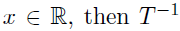is differentiable and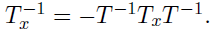Using the base equations we thus infer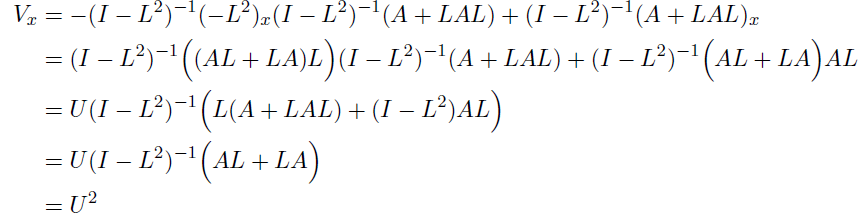Analogously, one checks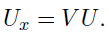In the same way one can calculate the derivative with respect to the time variable. Note that here the fact that [A, J] = 0 and {L, J} = 0 is crucial. We omit the proof.

Lemma 2. The derivative of the operator-valued function U = U(x, t) given in (2.2) with respect to t is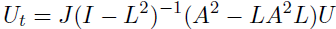Lemma 3. For the operator-functions U, V in (2.2), (2.3), the following identity holds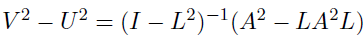(2.4)

Proof. We need the following auxiliary identity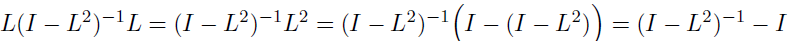which is applied in the third step of the succeeding calculation to replace the terms in the first and in the last large brackets.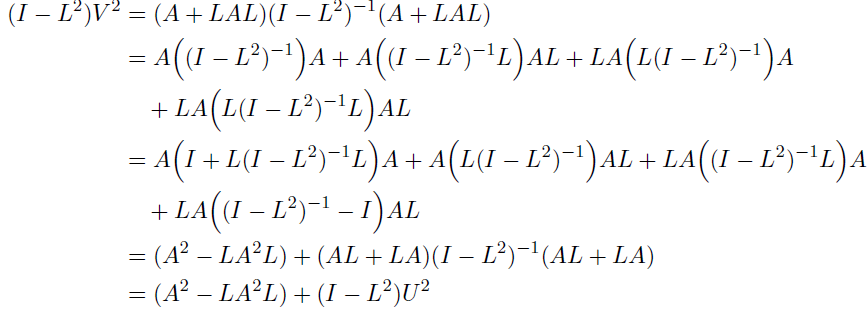Proof of Theorem 3. Using Lemma 1 we get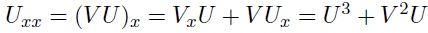Thus, applying successively Lemma 3 and Lemma 2,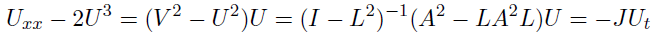Proof of Theorem 1. We obtain Theorem 1 by applying Theorem 3 to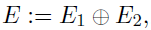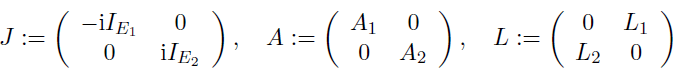#### Solution formulas for the NLS

Next we explain how Theorem 1 can be used to extract explicit solution formulas for the scalar NLS system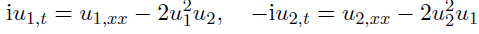(3.1)

To formulate our result we recall that a one-dimensional operator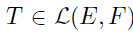can be written as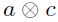with appropriate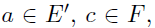where the map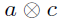is defined by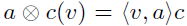(and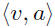denotes the evaluation of the functional a on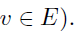Proposition 1. Let E1, E2 be Banach spaces and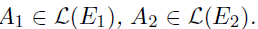Assume that there are operators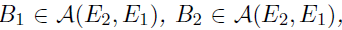belonging to a quasi-Banach ideal A admitting a continuous determinant ±, which satisfy the one-dimensionality conditions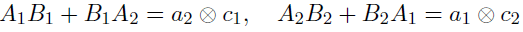(3.2)

with functionals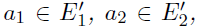and vectors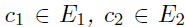and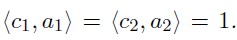Then a solution of the NLS system (3.1) is given by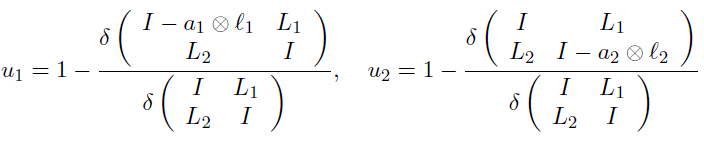(3.3)

with the operator-functions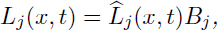the vector-functions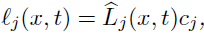where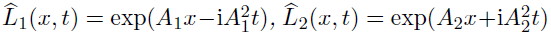provided the denominator does not vanish.

Remark 1. We want to stress that the one-dimensionality condition (3.2) can always be met provided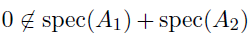(Minkowski sum), see also . The normalization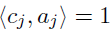is only chosen for convenience. It suffices to assume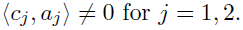Proof. The main argument of the proof is contained in the Step 2 where a solution of the scalar system is constructed from a solution of the non-abelian system by cross-evaluation.

Step 1: Applying Theorem 1, it can be immediately checked that the operator-functions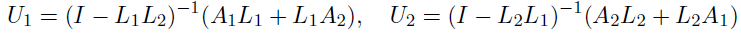solve the non-abelian NLS system (1.1).

Step 2: As a consequence of the one-dimensionality condition (3.2),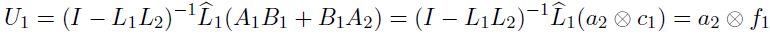where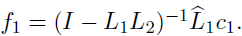Similarly,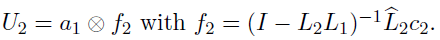We now show that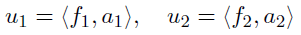(3.4)

solve (3.1). Indeed, evaluating the first equation of the operator system (1.1) on the vector c2, we obtain the vector-equation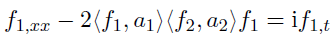Applying the functional a1, we get the first equation of the system (3.1). Similarly for the second equation of (3.1).

Step 3: It remains to verify the solution formula in terms of the determinant available on the underlying quasi-Banach ideal. To this end we first note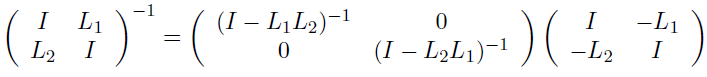Using the multiplicity property of a determinant and the fact that on the finite-dimensional operators the generalized determinant coincides with the standard determinant2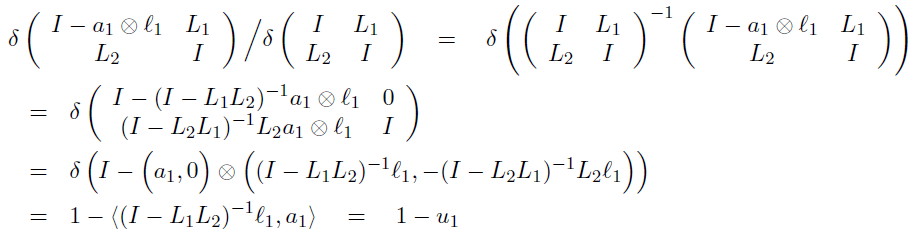and similarly for the other identity in (3.3).

#### Countable superposition of solitons

As an application we study solutions of the NLS arising from our solution formula by plugging in diagonal operators on sequence spaces. The resulting solution class describes the superposition of countably many solitons.

Choose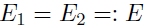to be one of the classical sequence spaces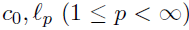and, for a given bounded sequence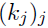with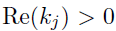for all j, we define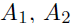to be the diagonal operators generated by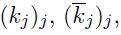respectively.

Let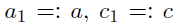be sequences satisfying the growth condition (1.3) and set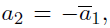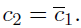Then the one-dimensionality condition (3.2) can be solved explicitly by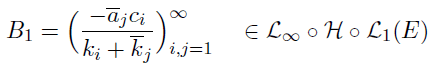and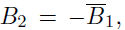where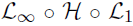denotes the quasi-Banach ideal of operators factorizing through first an L1-space, then a Hilbert space, and finally an L-space.

Indeed,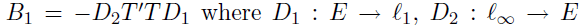are the diagonal operators generated by the sequences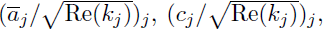and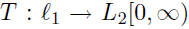defined on the standard basis by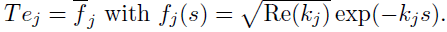Since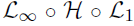admits a continuous (even spectral) determinant det¸ , the solution formula of Proposition 1 can be applied. Moreover one can check that the particular choices above guarantee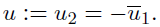This yields Theorem 2.

Remark 2. The results of this section can be easily generalized to construct also countable superpositions of multipole solutions of the NLS. Moreover it can be shown that all these solutions are globally regular. For details see .

#### Acknowledgement

I would like to thank the referee for careful reading and valuable remarks leading to a more suggestive presentation of the main results.

1Presented at the 3rd Baltic-Nordic Workshop “Algebra, Geometry, and Mathematical Physics“, G¨oteborg, Sweden, October 11–13, 2007.

2in particular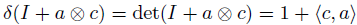for one-dimensional endomorphisms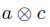#### References

Select your language of interest to view the total content in your interested language

### Article Usage

• Total views: 11886
• [From(publication date):
September-2008 - Dec 09, 2019]
• Breakdown by view type
• HTML page views : 8063Can't read the image? click here to refresh